Next: 2 Transformations of Data Up: 5 Applications of Matrix Previous: 5 Applications of Matrix   Index

## 1 Calculation of Covariance Matrix from Data Matrix

Suppose we have a data matrixwith rows corresponding to subjects and columns corresponding to variables. We can calculate a mean for each variable and replace the data matrix with a matrix of deviations from the mean. That is, each elementis replaced by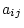whereis the mean of the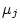variable. Let us call the new matrix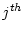. The covariance matrix is then simply calculated as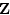where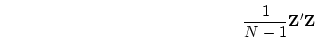is the number of subjects. For example, suppose we have the following data: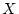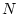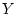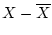1 2 -2 -4 2 8 -1 2 3 6 0 0 4 4 1 -2 5 10 2 4

So the matrix of deviations from the mean is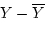and therefore the covariance matrix of the observations isThe diagonal elements of this matrix are the variances of the variables, and the off-diagonal elements are the covariances between the variables. The standard deviation is the square root of the variance (see Chapter 2). The correlation is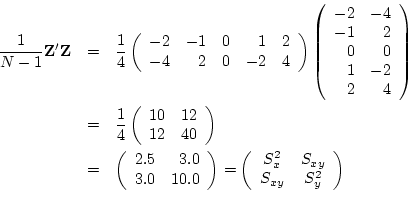In general, a correlation matrix may be calculated from a covariance matrix by pre- and post-multiplying the covariance matrix by a diagonal matrix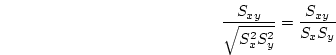in which each diagonal element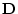is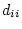, i.e., the reciprocal of the standard deviation for that variable. Thus, in our two variable example, we have: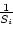Next: 2 Transformations of Data Up: 5 Applications of Matrix Previous: 5 Applications of Matrix   Index
Jeff Lessem 2002-03-21